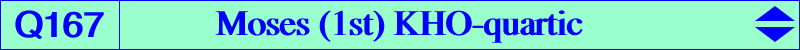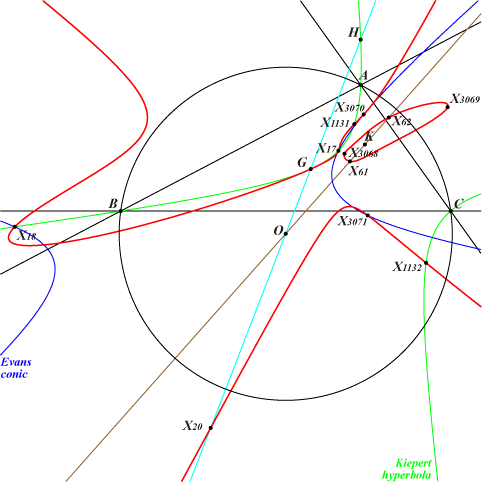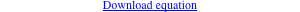too complicated to be written here. Click on the link to download a text file.X(2), X(17), X(18), X(20), X(61), X(62), X(1131), X(1132), X(3068), X(3069), X(3070), X(3071), X(39641), X(39642) X(39641), X(39642) are the imaginary foci of the Brocard inellipse, on the Brocard axis and the Kiepert hyperbolaQ167 is a KHO-curve, see K1191 and CL075 for information. See also Q073, another KHO-quartic. The KHO-equation is : 2x^4 - x^2 ( 94y^2 - 59yz + 12z^2) + (2y - z) (2y + z) (66y^2 - 101yz + 54z^2) = 0. Other KHO-centers on the curve : (±88 √3, 45, 86), (±80 √3, 99, 38), (240, -29, 22), (240, 29, -22). *** Peter Moses also found several other similar KHO-quartics listed in the table below.KHO-equation X(i) on the curve for these i 2x^4 + 3x^2 y (10y - 9z) - 9 (y -z) (2y - z) (6y^2 - 3yz + 2z^2) = 0 see Q168 2x^4 - 3x^2 y (10y - z) + 3 (2y -z) (2y + z) (6y^2 - 7yz + 6z^2) = 0 2, 17, 18, 20, 371, 372, 1131, 1132, 1587, 1588, 3068, 3069, 3070, 3071, 39641, 39642 2x^4 + 3x^2 y (46y - 27z) - 9 (2y -z) (2y + z) (12y^2 - 3yz - 2z^2) = 0 2, 17, 18, 20, 371, 372, 485, 486, 3068, 3069, 3070, 3071, 39641, 39642